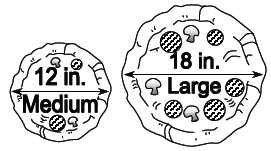### Home > MC2 > Chapter 6 > Lesson 6.2.5 > Problem6-118

6-118.

Whole pizzas are described by the length of their diameters. For example, a $12$-inch pizza has a diameter of $12$ inches.Stan the Pizza Man told a customer that a $12^{\prime\prime}$ medium pizza costs $10$ and an $18^{\prime\prime}$ large pizza costs $16$. Which pizza is the better deal for the customer (that is, which one costs less per square inch)? You may use a calculator, but you must show your work.

To find the best cost per square inch, we must first find the area of each pizza.

Using the formula for the area of a circle, $r^2π$:
Medium pizza: $6^2π = 36π = 113.04\text{ in}^2$.
Large pizza: $9^2π = 81π = 254.34\text{ in}^2$.

Remember, $12$ in. and $18$ in. are the diameters of the pizzas.

Now with the area, we can determine the price per square inch of pizza. We can convert to cents for a better indicator of price.

Medium pizza: $10 = 1000$ cents

$\frac{1000\text{ cents}}{113.04\text{ in^2 }}\approx9$ cents per square inch

Large pizza: $16 = 1600$ cents

$\frac{1600\text{ cents}}{254.34\text{ in^2}}\approx6$ cents per square inch